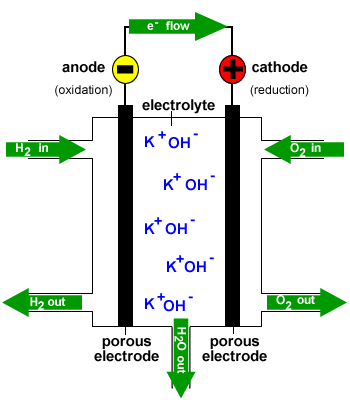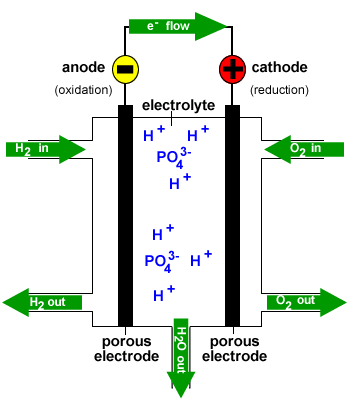Fuel Cells Chemistry Tutorial

Key Concepts

• A fuel cell is a galvanic (voltaic) cell in which the reactants are continuously fed into the cell as the cell produces electricity.
• Fuel cells are more efficient at converting the chemical energy of fuels into electrical energy compared to the combustion of the same fuel in air.
• In general, a fuel cell produces about 0.7 volts.
• A generalised fuel cell is shown below:

 e- → electrolyte fuel → ← oxidant ← excess fuel excess oxidant → wasteproducts↓ porous anode porous cathode

• At the anode:
The fuel is the reductant (reducing agent).

The fuel is the substance that will be oxidised.

The oxidation of the fuel produces electrons.

The anode is negative.

Oxidation occurs at the anode.

Electrons flow from the anode (-) to the cathode (+)

• At the cathode:
The oxidant (oxidizing agent) is the substance that will be reduced.

The reduction of the oxidant consumes electrons.

The cathode is positive.

Reduction occurs at the cathode.

• The porous electrodes:
(a) conduct electrons

(b) physically separate the fuel and the oxidant

(c) allow contact between the reactants and the electrolyte

(d) act as catalysts for the oxidation and reduction reactions

• Maximum voltage that can be delivered by the cell during discharge is equal to the electrode potential for the spontaneous redox reaction.

Under standard conditions, maximum voltage during discharge = Eo(redox)

No ads = no money for us = no free stuff for you!

Example: Alkaline Fuel Cell

Alkaline fuel cells, or Bacon fuel cells, consume hydrogen gas and pure oxygen gas to produce electricity, water and heat.

Alkaline fuel cells have been used to supply electricity and drinking water onboard America's spacecraft.• Electrolyte:
hot KOH(aq)
• Anode:
Porous carbon electrode with catalyst in contact with H2(g)

Fuel (reductant): hydrogen gas

H2(g) is oxidized at the anode:

H2(g) + 2OH-(aq) → 2H2O(l) + 2e-

Electrons are produced at the anode.

Anode is negative (-).

Electrons flow from the anode (-) to the cathode (+) through connecting wires.

H2O migrates from anode to cathode through electrolyte.

• Cathode :
Porous carbon cathode with catalyst in contact with O2(g)

Oxidant : oxygen gas

O2(g) is reduced at the cathode:

O2(g) + 2H2O(l) + 4e- → 4OH-(aq)

Electrons are consumed at the cathode.

Cathode is positive (+)

Half the water produced at the anode is consumed at the cathode.

OH- migrates from cathode to anode through electrolyte.

• Overall fuel cell reaction:

 2× anode: 2H2(g) + 4OH-(aq) → 42H2O(l) + 4e- cathode: O2(g) + 2H2O(l) + 4e- → 4OH-(aq) overall: 2H2(g) + O2(g) → 2H2O(l) E(cell) ≈ 0.7 V This excess H2O(l) is removed from the fuel cell so that the concentration of KOH(aq) is maintained.

Do you know this?

Play the game now!

Example: Phosphoric Acid Fuel Cell

Phosphoric acid fuel cells consume hydrogen gas and oxygen gas from air to produce electricity, water and heat.

Phosphoric acid fuel cells can be used as stationary power generators and have also been used in buses.• Electrolyte:
hot concentrated phosphoric acid
• Anode:
Porous carbon electrode with catalyst in contact with H2(g)

Fuel (reductant): hydrogen gas

H2(g) is oxidized at the anode:

H2(g) → 2H+(aq) + 2e-

Electrons are produced at the anode.

Anode is negative (-).

Electrons flow from the anode (-) to the cathode (+) through connecting wires.

H+ migrates from anode to cathode through electrolyte.

• Cathode :
Porous carbon cathode with catalyst in contact with O2(g)

Oxidant : oxygen gas

O2(g) is reduced at the cathode:

O2(g) + 4H+(aq) + 4e- → 2H2O(l)

H+ produced at the anode is consumed at the cathode.

Electrons are consumed at the cathode.

Cathode is positive (+)

OH- migrates from cathode to anode through electrolyte.

• Overall fuel cell reaction:

 2× anode: 2H2(g) → 4H+(aq) + 4e- cathode: O2(g) + 4H+(aq) + 4e- → 2H2O(l) overall: 2H2(g) + O2(g) → 2H2O(l) E(cell) ≈ 0.7 V H2O(l) produced at the cathode is removed so that the concentration of H3PO4 is maintained.

Do you understand this?

Take the test now!

Example: Direct Methanol Fuel Cell

Direct methanol fuel cells consume methanol, CH3OH, and oxygen to produce electricity, heat, carbon dioxide and water.
A proton exchange membrane is used in place of the electrolyte which allows H+ produced at the anode to migrate to the cathode.

Methanol fuel cells can be used to power mobile phones, digital cameras and laptop computers.

 e- → membrane methanol → ← oxygen ← methanol oxygen → CO2H2O↓ anode  cathode

• Anode:
A catalyst, often platinum, in contact with CH3OH(aq)

Fuel (reductant): 1 mol L-1 CH3OH(aq)

CH3OH(aq) is oxidized at the anode:

CH3OH(aq) + H2O(l) → 6H+(aq) + CO2(g) + 6e-

Electrons are produced at the anode.

Anode is negative (-).

Electrons flow from the anode (-) to the cathode (+) through connecting wires.

H+ migrates from anode to cathode through the membrane.

• Cathode :
A catalyst, often platinum, in contact with O2(g)

Oxidant : oxygen gas

O2(g) is reduced at the cathode:

O2(g) + 4H+(aq) + 4e- → 2H2O(l)

H+ produced at the anode is consumed at the cathode.

Electrons are consumed at the cathode.

Cathode is positive (+)

H2O migrates from cathode to anode through the membrane.

• Overall fuel cell reaction:

 2× anode: 2CH3OH(aq) + 2H2O(l) → 12H+(aq) + 2CO2(g) + 12e- 3× cathode: 3O2(g) + 12H+(aq) + 12e- → 64H2O(l) overall: 2CH3OH(aq) + 3O2(g) → 2CO2(g) + 4H2O(l) E(cell) ≈ 0.7 V H2O(l) and CO2(g) produced at the cathode are removed from the fuel cell.

Can you apply this?

Join AUS-e-TUTE!

Take the exam now!

Example: Direct Ethanol Fuel Cell

Direct ethanol fuel cells consume ethanol, C2H5OH, and oxygen to produce electricity, heat, carbon dioxide and water.
A proton exchange membrane is used in place of the electrolyte which allows H+ produced at the anode to migrate to the cathode.

Ethanol fuel cells could one day be used to charge appliances such as mobile phones.

 e- → membrane ethanol → ← oxygen ← ethanol oxygen → CO2H2O↓ anode  cathode

• Anode:
A catalyst, often platinum, in contact with C2H5OH(aq)

Fuel (reductant): C2H5OH(aq)

C2H5OH(aq) is oxidized at the anode:

C2H5OH(aq) + 3H2O(l) → 12H+(aq) + 2CO2(g) + 12e-

Electrons are produced at the anode.

Anode is negative (-).

Electrons flow from the anode (-) to the cathode (+) through connecting wires.

H+ migrates from anode to cathode through the membrane.

• Cathode :
A catalyst, often platinum, in contact with O2(g)

Oxidant : oxygen gas

O2(g) is reduced at the cathode:

O2(g) + 4H+(aq) + 4e- → 2H2O(l)

H+ produced at the anode is consumed at the cathode.

Electrons are consumed at the cathode.

Cathode is positive (+)

H2O migrates from cathode to anode through the membrane.

• Overall fuel cell reaction:

 1× anode: C2H5OH(aq) + 3H2O(l) → 12H+(aq) + 2CO2(g) + 12e- 3× cathode: 3O2(g) + 12H+(aq) + 12e- → 63H2O(l) overall: C2H5OH(aq) + 3O2(g) → 2CO2(g) + 3H2O(l) E(cell) ≈ 0.7 V H2O(l) and CO2(g) produced at the cathode are removed from the fuel cell.

Can you apply this?

Join AUS-e-TUTE!

Take the exam now!